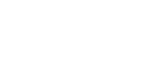## 归一化markmark

• 线性函数归一化(Min-Max Scaling)
• 0均值标准化(Z-Score Standardization)markmark

## 参数初始化问题

### 怎样初始化权重markmark

## 怎样初始化权重 二

`def large_weight_initializer(self):        # 初始化每层的偏置        self.biases = [np.random.randn(y, 1) for y in self.sizes[1:]]        # 初始化每层的权重        self.weights = [np.random.randn(y, x)                        for x, y in zip(self.sizes[:-1], self.sizes[1:])]`

`def default_weight_initializer(self):        # 初始化每层的偏置和之前一样        self.biases = [np.random.randn(y, 1) for y in self.sizes[1:]]        # 初始化每层的权重        self.weights = [np.random.randn(y, x)/np.sqrt(x)                        for x, y in zip(self.sizes[:-1], self.sizes[1:])]`markmark